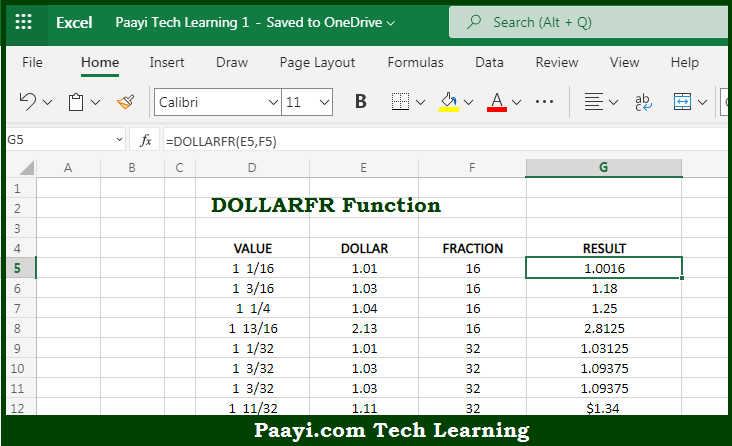# Learn How to Use Microsoft Excel DOLLARFR Function

Written by | 0 Comments | 593 Views

In this article, you will learn how to use the Microsoft Excel DOLLARFR function and its prime function in Microsoft Excel. You will also get to know the Microsoft Excel DOLLARFR function return value and syntax with the help of some examples.

Microsoft Excel DOLLARFR Function

The main purpose of the Microsoft Excel DOLLARFR function is to convert price to fractional notation. That implies, with the help of DOLLARFR function you can able to convert a dollar price in a regular decimal number format to a dollar price in a particular fractional notation used for securities where pricing is given to the nearest 1/8, 1/16, 1/32, etc. It should be noted that the DOLLARDE function does the opposite conversion. So, with the help of the DOLLARFR function, you can able to convert price to fractional notation.

Return Value of DOLLARFR Function

The return value will be the converted price to fractional notation.

Syntax of DOLLARFR Function

=DOLLARFR(decimal-dollar, fraction)

Where the arguments:

• decimal-dollar: This is the pricing as a normal decimal number.
• fraction: This is the denominator in the fractional unit. 8 = 1/8, 16 = 1/16, 32 = 1/32, etc.

## How to Use Microsoft Excel DOLLARFR Function?So we know that Microsoft Excel DOLLARFR function you can able to convert price to fractional notation. That implies, with the help of the DOLLARFR function you can able to convert a dollar price in a regular decimal number format to a dollar price in a particular fractional notation used for securities where pricing is given to the nearest 1/8, 1/16, 1/32, etc. It should be noted that the DOLLARDE function does the opposite conversion. So, with the help of the DOLLARFR function, you can able to convert price to fractional notation.